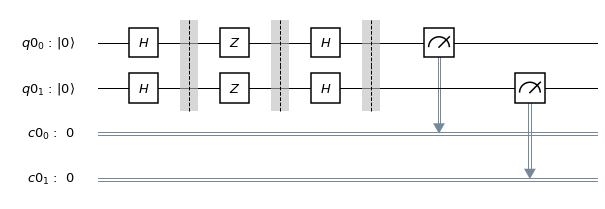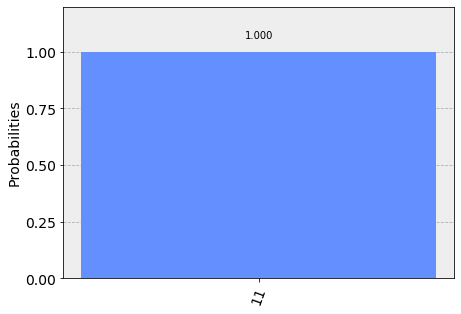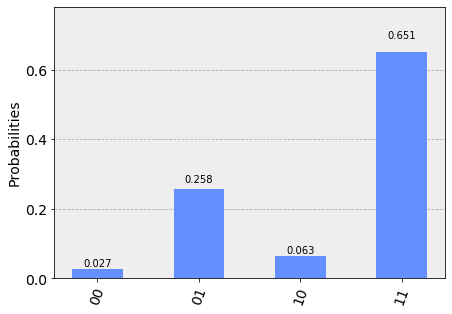# Bernstein-Vazirani Algorithm¶

In this section, we first introduce the Bernstein-Vazirani problem, and classical and quantum algorithms to solve it. We then implement the quantum algorithm using Qiskit, and run on a simulator and device.

## 1. Introduction ¶

The Bernstein-Vazirani algorithm, first introduced in Reference , can be seen as an extension of the Deutsch-Josza algorithm covered in the last section. It showed that there can be advantages in using a quantum computer as a computational tool for more complex problems compared to the Deutsch-Josza problem.

### 1a. Bernstein-Vazirani Problem ¶

We are again given a hidden function Boolean $f$, which takes as as input a string of bits, and returns either $0$ or $1$, that is:

$f(\{x_0,x_1,x_2,...\}) \rightarrow 0 \textrm{ or } 1 \textrm{ where } x_n \textrm{ is }0 \textrm{ or } 1$.

Instead of the function being balanced or constant as in the Deutsch-Josza problem, now the function only returns $1$ for a particular $n$ length bit string $s$, and for all other inputs it returns $0$. In other words, for each input $x$, $f(x) = s \cdot x \, \text{(mod 2)}$. We are expected to find $s$.

### 1b. Bernstein-Vazirani Algorithm ¶

#### Classical Solution¶

Classically, the oracle returns $f_s(x) = s \cdot x \mod 2$ given an input $x$. Thus, the hidden bit string $s$ can be revealed by querying the oracle with $x = 1, 2, \ldots, 2^i, \ldots, 2^{n-1}$, where each query reveals the $i$-th bit of $s$ (or, $s_i$). For example, with $x=1$ one can obtain the least significant bit of $s$, and so on. This means we would need to call the function $f_s(x)$ $n$ times.

#### Quantum Solution¶

Using a quantum computer, we can solve this problem with 100% confidence after only one call to the function $f(x)$. The quantum Bernstein-Vazirani algorithm to find the hidden integer is very simple: (1) start from a $|0\rangle^{\otimes n}$ state, (2) apply Hadamard gates, (3) query the oracle, (4) apply Hadamard gates, and (5) measure, generically illustrated below:The correctness of the algorithm is best explained by looking at the transformation of a quantum register $|a \rangle$ by $n$ Hadamard gates, each applied to the qubit of the register. It can be shown that:

$$|a\rangle \xrightarrow{H^{\otimes n}} \frac{1}{\sqrt{2^n}} \sum_{x\in \{0,1\}^n} (-1)^{a\cdot x}|x\rangle.$$

In particular, when we start with a quantum register $|0\rangle$ and apply $n$ Hadamard gates to it, we have the familiar quantum superposition:

$$|0\rangle \xrightarrow{H^{\otimes n}} \frac{1}{\sqrt{2^n}} \sum_{x\in \{0,1\}^n} |x\rangle,$$

which is slightly different from the Hadamard transform of the reqister $|a \rangle$ by the phase $(-1)^{a\cdot x}$.

Now, the quantum oracle $f_a$ returns $1$ on input $x$ such that $a \cdot x \equiv 1 \mod 2$, and returns $0$ otherwise. This means we have the following transformation:

$$|x \rangle \xrightarrow{f_a} | x \rangle = (-1)^{a\cdot x} |x \rangle.$$

The algorithm to reveal the hidden integer follows naturally by querying the quantum oracle $f_a$ with the quantum superposition obtained from the Hadamard transformation of $|0\rangle$. Namely,

$$|0\rangle \xrightarrow{H^{\otimes n}} \frac{1}{\sqrt{2^n}} \sum_{x\in \{0,1\}^n} |x\rangle \xrightarrow{f_a} \frac{1}{\sqrt{2^n}} \sum_{x\in \{0,1\}^n} (-1)^{a\cdot x}|x\rangle.$$

Because the inverse of the $n$ Hadamard gates is again the $n$ Hadamard gates, we can obtain $a$ by

$$\frac{1}{\sqrt{2^n}} \sum_{x\in \{0,1\}^n} (-1)^{a\cdot x}|x\rangle \xrightarrow{H^{\otimes n}} |a\rangle.$$

## 2. Example ¶

Let's go through a specific example for $n=2$ qubits and a secret string $s=11$. Note that we are following the formulation in Reference  that generates a circuit for the Bernstein-Vazirani quantum oracle using only one register.

1. The register of two qubits is initialized to zero: $$\lvert \psi_0 \rangle = \lvert 0 0 \rangle$$
2. Apply a Hadamard gate to both qubits: $$\lvert \psi_1 \rangle = \frac{1}{2} \left( \lvert 0 0 \rangle + \lvert 0 1 \rangle + \lvert 1 0 \rangle + \lvert 1 1 \rangle \right)$$
3. For the string $s=11$, the quantum oracle can be implemented as $\text{Q}_f = Z_{1}Z_{2}$: $$\lvert \psi_2 \rangle = \frac{1}{2} \left( \lvert 0 0 \rangle - \lvert 0 1 \rangle - \lvert 1 0 \rangle + \lvert 1 1 \rangle \right)$$
4. Apply a Hadamard gate to both qubits: $$\lvert \psi_3 \rangle = \lvert 1 1 \rangle$$
5. Measure to find the secret string $s=11$

## 3. Qiskit Implementation ¶

We now implement the Bernstein-Vazirani algorithm with Qiskit for a two bit function with $s=11$.

In :
# initialization
import matplotlib.pyplot as plt
%matplotlib inline
import numpy as np

# importing Qiskit
from qiskit import IBMQ, BasicAer
from qiskit.providers.ibmq import least_busy
from qiskit import QuantumCircuit, ClassicalRegister, QuantumRegister, execute

# import basic plot tools
from qiskit.tools.visualization import plot_histogram


We first set the number of qubits used in the experiment, and the hidden integer $s$ to be found by the algorithm. The hidden integer $s$ determines the circuit for the quantum oracle.

In :
nQubits = 2 # number of physical qubits used to represent s
s = 3       # the hidden integer

# make sure that a can be represented with nqubits
s = s % 2**(nQubits)


We then use Qiskit to program the Bernstein-Vazirani algorithm.

In :
# Creating registers
# qubits for querying the oracle and finding the hidden integer
qr = QuantumRegister(nQubits)
# bits for recording the measurement on qr
cr = ClassicalRegister(nQubits)

bvCircuit = QuantumCircuit(qr, cr)
barriers = True

# Apply Hadamard gates before querying the oracle
for i in range(nQubits):
bvCircuit.h(qr[i])

# Apply barrier
if barriers:
bvCircuit.barrier()

# Apply the inner-product oracle
for i in range(nQubits):
if (s & (1 << i)):
bvCircuit.z(qr[i])
else:
bvCircuit.iden(qr[i])

# Apply barrier
if barriers:
bvCircuit.barrier()

#Apply Hadamard gates after querying the oracle
for i in range(nQubits):
bvCircuit.h(qr[i])

# Apply barrier
if barriers:
bvCircuit.barrier()

# Measurement
bvCircuit.measure(qr, cr)

Out:
<qiskit.circuit.instructionset.InstructionSet at 0x1276416d8>
In :
bvCircuit.draw(output='mpl')

Out:### 3a. Experiment with Simulators ¶

We can run the above circuit on the simulator.

In :
# use local simulator
backend = BasicAer.get_backend('qasm_simulator')
shots = 1024
results = execute(bvCircuit, backend=backend, shots=shots).result()


Out:We can see that the result of the measurement is the binary representation of the hidden integer $3$ $(11)$.

### 3b. Experiment with Real Devices ¶

We can run the circuit on the real device as below.

In :
# Load our saved IBMQ accounts and get the least busy backend device with less than or equal to 5 qubits
provider = IBMQ.get_provider(hub='ibm-q')provider.backends()
backend = least_busy(provider.backends(filters=lambda x: x.configuration().n_qubits <= 5 and
not x.configuration().simulator and x.status().operational==True))
print("least busy backend: ", backend)

least busy backend:  ibmqx2

In :
# Run our circuit on the least busy backend. Monitor the execution of the job in the queue
from qiskit.tools.monitor import job_monitor

shots = 1024
job = execute(bvCircuit, backend=backend, shots=shots)

job_monitor(job, interval = 2)

Job Status: job has successfully run

In :
# Get the results from the computation
results = job.result()


Out:As we can see, most of the results are $11$. The other results are due to errors in the quantum computation.

## 4. Problems ¶

1. The above implementation of Bernstein-Vazirani is for a secret bit string of $s = 11$. Modify the implementation for a secret string os $s = 1011$. Are the results what you expect? Explain.
2. The above implementation of Bernstein-Vazirani is for a secret bit string of $s = 11$. Modify the implementation for a secret string os $s = 1110110101$. Are the results what you expect? Explain.

## 5. References ¶

In :
import qiskit
qiskit.__qiskit_version__

Out:
{'qiskit': '0.10.4',
'qiskit-terra': '0.8.2',
'qiskit-ignis': '0.1.1',
'qiskit-aer': '0.2.1',
'qiskit-ibmq-provider': '0.2.2',
'qiskit-aqua': '0.5.1'}